Next: 3.3.4 Effective Carrier Mass Up: 3.3 Band-Structure Previous: 3.3.2 Bandgap Offsets

Subsections

## 3.3.3 Bandgap Narrowing

Bandgap narrowing (BGN) is one of the crucial heavy-doping effects to be considered for bipolar devices. In MINIMOS-NT the use of BGN model is optional. The model of Slotboom  is widely used in case of silicon.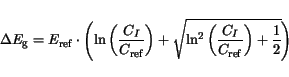(3.73)

The shifts of the conduction and valence band edges are calculated by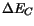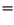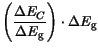(3.74)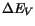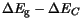(3.75)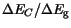gives the part of the total BGN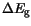which is contributed to the conduction band. The default parameter values are summarized in the following table.

Table 3.15: Parameter values for modeling the bandgap narrowing
 Material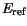[eV]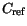[cm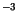]Si 0.009 1e17 0.5

Using the physically-based approach from , a new analytical BGN model was developed. It is applicable to compound semiconductors  and considers the semiconductor material and the dopant species for arbitrary finite temperatures. In this section a comparison with experimental data and other existing models is presented and study of BGN in III-V group semiconductors is performed.

### 3.3.3.1 Dopant-Dependent Bandgap Narrowing

Despite of many papers dedicated on BGN in semiconductors, the optimal balance between accuracy of the results and simplicity of model implementation seems had not been achieved. Commercial device simulators, such as ATLAS  , DESSIS , and MEDICI , use the logarithmic fit models for BGN in Si from [133,135,136,137] which are simple to implement, but deliver non-physical values below and above definite doping levels. Furthermore, the functional form of models for Si is used for models for other materials (e.g. III-V compounds ) or the BGN effect is left completely ignored. Comparisons of these models are shown in Fig. 3.21. The physical limit our model offers (0 meV for undoped materials), the physically sound explanation of some existing effects it gives, the ability to treat various semiconductor materials and dopants, and the simplicity of the model, make it very applicable for device simulation purposes.

### 3.3.3.2 Physical background of the new model

The basic assumption in our model is that BGN is a result of five types of many-body interactions (electron-electron, electron-impurity, hole-hole, hole-impurity, and electron-hole). The electron-impurity contribution is assumed to be dominant at high doping concentrations. Though BGN is very difficult to model rigorously due to multiple carrier interactions one can approximate the energy shift to first order by the classical self-energy of the electron in the field of an ionized impurity . Thus one obtains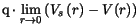(3.76)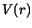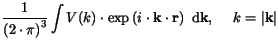(3.77)

Here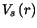anddenote the screened and unscreened Coulomb potentials of the impurity, respectively. (3.76) represents the change in the electrostatic energy of the impurity before and after the electron gas redistribution. If the potential of a point-like impurity is assumed, the Fourier transform of the scattering potential is expressed by (3.78), where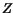and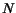are the atomic number and the number of electrons of a given material,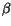denotes the inverse Thomas-Fermi length, and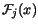is the Fermi integral of order. This approach leads to a simple BGN model  given by (3.80).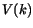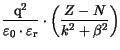(3.78)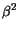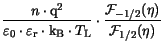(3.79)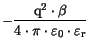(3.80)

Removing the point-charge approximation yields a refined model. The charge density of the impurity can be accounted for by an atomic form factor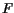. Following the work  the impurity potential takes the form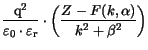(3.81)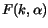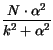(3.82)

Solving (3.77) using (3.81) and (3.82), and then replacing V(r) in (3.76), leads to the final expression for the energy shift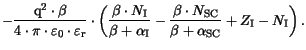(3.83)

The subscripts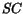and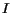refer to a semiconductor and impurity, respectively.andare the atomic number and the number of electrons of a given material.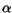can be interpreted as size parameters of the electron charge density and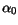is the Bohr radius. They are expressed as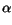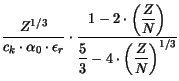(3.84)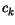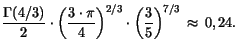(3.85)

The size parameteruses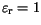, which is the most pessimistic estimation, since it is still not clarified which value forin the range between 1 and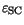is valid at microscopic level. Even though the influence of the dopant type is reduced to minimum this way, our model still delivers appropriate results at 300 K in agreement with experiment  (see Fig. 3.22).

The temperature dependence of the BGN in Si is shown in Fig. 3.23. Neglecting of the stronger BGN at low temperatures, especially for doping levels of about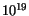cm, may result in an error of about 50%. Thereby, even larger errors might be introduced into the simulation results, with respect to the electrical device characteristics. In the case of III-V semiconductors our model delivers a comparatively weaker BGN temperature dependence (see Fig. 3.24). Similar observations were reported in the case of p-GaAs in , . Thus, according to our knowledge, our BGN model is the first theoretically derived model predicting different shifts for various dopant species and taking temperature into account.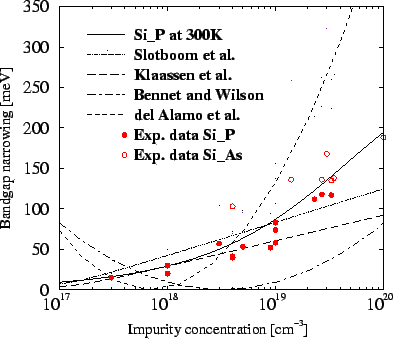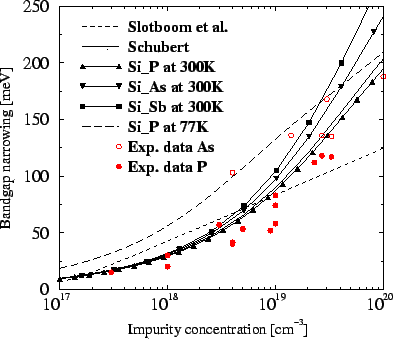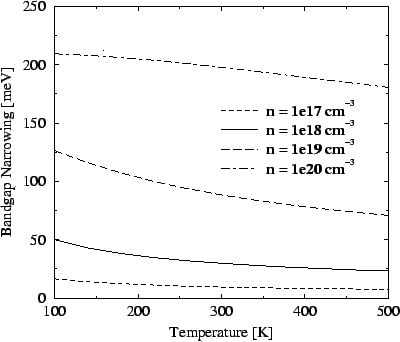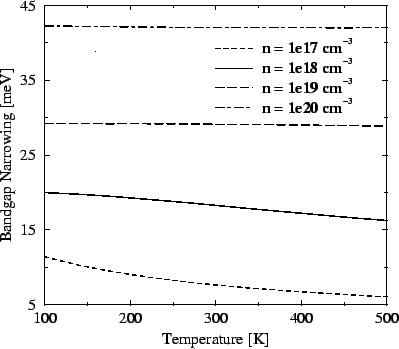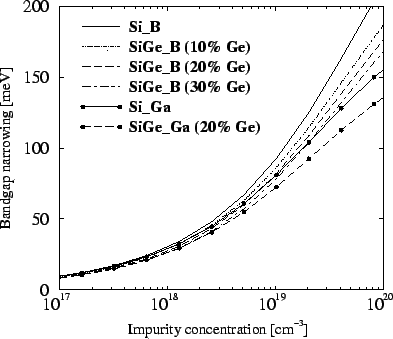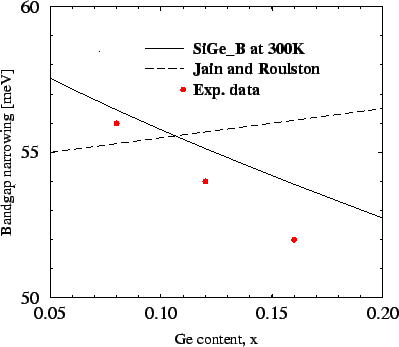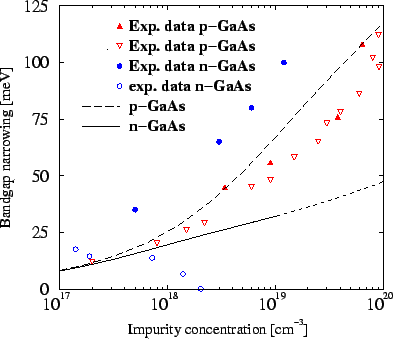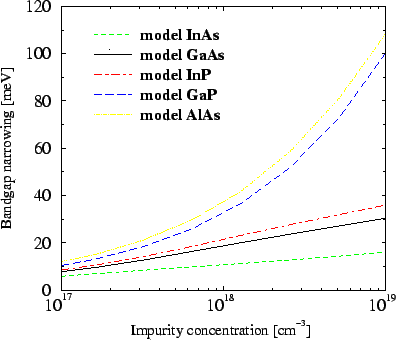### 3.3.3.3 Extending the new model to semiconductor alloys

The model extends its validity also for alloy semiconductors by material composition dependent relative effective masses for electrons and holes, on the one hand, and permittivity, on the other hand. In Fig. 3.25 the results for boron and gallium doped Si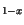Ge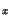for different Ge contents are presented. This is even better illustrated in Fig. 3.26 where the BGN versus material composition in SiGeis compared to another model proposed by Jain and Roulston  suggesting increased BGN. The decrease of the BGN with increase of the Ge fraction was already experimentally observed in , . Our theoretical approach explains this effect by the decreased valence band density of states and increase of the relative permittivity in the SiGe alloy.

In the case of p-type GaAs good agreement with experimental data ,  is obtained. The few available experimental data for n-type GaAs suggest sometimes lower  (open triangles in Fig. 3.27) values for BGN and more often higher  (filled triangles) than our model delivers. This confirms the importance of modeling BGN in III-V semiconductors, instead of leaving this effect unconsidered, which is the case with most device simulators.

Experiments showed higher BGN in n-InP than in n-GaAs . Higher conduction band density of states and lower relative permittivity explain the expected higher values for BGN in AlAs and GaP (Fig. 3.28) than in InP, GaAs, and InAs. The parameter values are taken from . The model is physics-based and contains no free parameters except the ratioused in (3.74).Next: 3.3.4 Effective Carrier Mass Up: 3.3 Band-Structure Previous: 3.3.2 Bandgap Offsets
Vassil Palankovski
2001-02-28